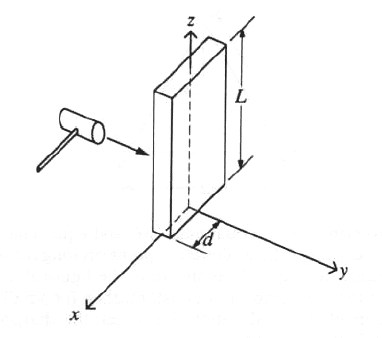GR 8677927796770177 | # Login | Register

GR9277 #82
Problem
 GREPhysics.NET Official Solution Alternate Solutions
\prob{82}A thin plate of mass M, length L, and width 2d is mounted vertically on a frictionless axle along the z-axis, as shown above. Initially the object is at rest. It is then tapped with a hammer to provide a torque , which produces an angular impulse about the z-axis of magnitude . What is the angular speed of the plate about the z-axis after the tap?

Mechanics}Torque

The problem gives , but . Thus, .

The moment of inertia of a plate about the z-axis is just . Plug this into to get choice (D).Alternate Solutions
 dberger82016-08-19 20:19:40 A lot like jmason86, I arrived at the answer a slightly different way. . Reply to this commentjmason862009-09-03 19:36:44 I arrived at another way. By analogy: and in the rotational system you get instead. Since the initial angular velocity was 0, the delta just becomes I = H. Some simple algebra and bam. Reply to this commentdberger8
2016-08-19 20:19:40
A lot like jmason86, I arrived at the answer a slightly different way. .asa1985
2011-10-06 09:43:46
Torque=dL/dt;
where L is Angular Momentum;

Then H= Troque dt
H= dL
H=L
As we know L= Iw

w=L/Ikroner
2009-09-26 18:13:21
We know will depend on I. The moment of inertia of a linear object like this is going to have a factor of 3 in it somewhere in terms of the mass and length, and there's no way for a factor of 3 to enter into the calculation elsewhere to cancel it out. That leaves choice (D).jmason86
2009-09-03 19:36:44
I arrived at another way.
By analogy: and in the rotational system you get instead. Since the initial angular velocity was 0, the delta just becomes I = H. Some simple algebra and bam.Anastomosis
2008-04-09 20:51:25
Although the test book doesn't give the moment of inertia for a plate, it does give it for a rod--if you look at it, a plate is just a thick rod, extended in the z-direction (the direction that has no bearing on the moment of inertia).

Anyway, for a rod is , where is just equal to , or in the general case.

So,FA
2007-04-13 00:29:06
typo alert. there should be no 2 in the denominator of the answer in D)i3taesun
2007-04-11 01:25:54
Like I=Fdt=delta P, H=torque dt=delta L.
Therefore, we should know the changing quantity of angular momentum.cherianjudy
2006-11-03 16:11:02jcain6
2005-11-23 07:00:05
I think w = at not a/t. This is why w = H/I right?
 yosun2005-11-23 15:02:18 jcain6: thanks for the typo-alert; it has been corrected. (while typing up this solution, my pinky was evidently slash-key happy.)LaTeX syntax supported through dollar sign wrappers $, ex.,$\alpha^2_0$produces . type this... to get...$\int_0^\infty\partial\Rightarrow\ddot{x},\dot{x}\sqrt{z}\langle my \rangle\left( abacadabra \right)_{me}\vec{E}\frac{a}{b}\$